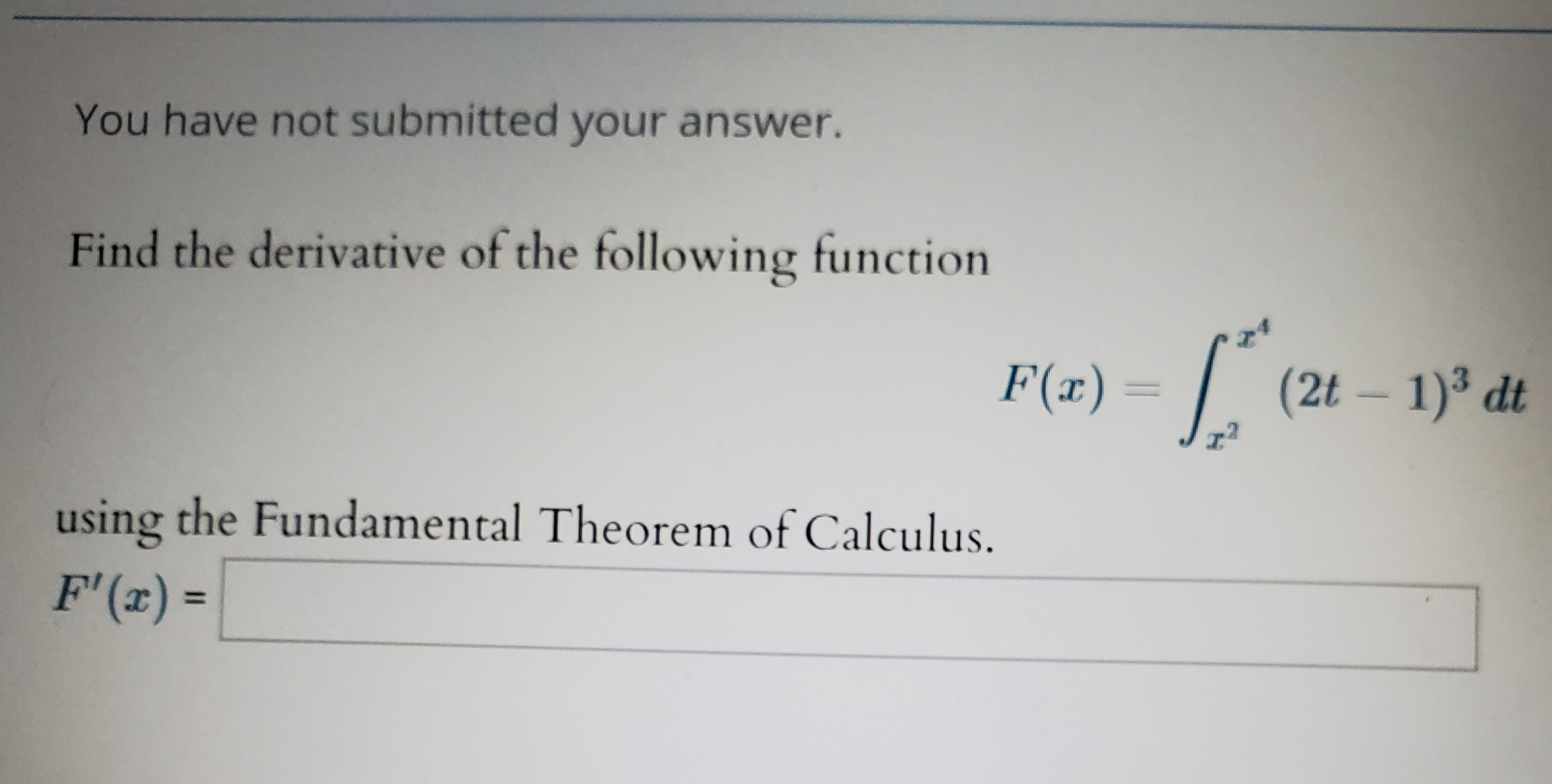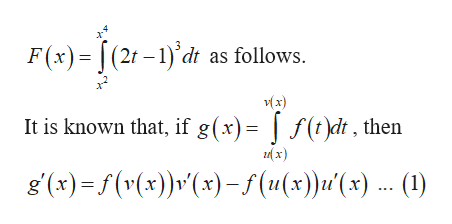# You have not submitted your answer.Find the derivative of the following functionF(a)(2t 1) dtusing the Fundamental Theorem of Calculus.F' (r)=

Question
10 viewshelp_outlineImage TranscriptioncloseYou have not submitted your answer. Find the derivative of the following function F(a)(2t 1) dt using the Fundamental Theorem of Calculus. F' (r) = fullscreen
check_circle

Step 1

Use fundamental theorem of calculus and com...help_outlineImage TranscriptioncloseF(x)= (2r-1) dt as follows. x) It is known that, if g(x) f(t)dt, then g (x)f(v(x)v(x)-f(u(x))u'(x) .. (1) fullscreen

### Want to see the full answer?

See Solution

#### Want to see this answer and more?

Solutions are written by subject experts who are available 24/7. Questions are typically answered within 1 hour.*

See Solution
*Response times may vary by subject and question.
Tagged in
MathCalculus

### Other